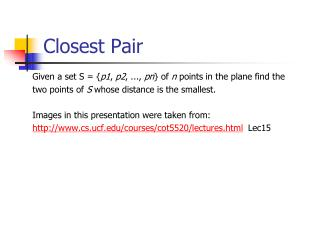DownloadDownload PresentationClosest Pair

# Closest Pair

Télécharger la présentation## Closest Pair

- - - - - - - - - - - - - - - - - - - - - - - - - - - E N D - - - - - - - - - - - - - - - - - - - - - - - - - - -
##### Presentation Transcript

1. Closest Pair Given a set S = {p1, p2, ..., pn} of n points in the plane find the two points of S whose distance is the smallest. Images in this presentation were taken from: http://www.cs.ucf.edu/courses/cot5520/lectures.html  Lec15

2. Closest Pair – Naïve Algorithm Pseudo code for each pt iS for each pt jS and i<>j { compute distance of i, j if distance of i, j < min_dist min_dist = distance i, j } return min_dist • Time Complexity– O(n2) • Can we do better?

3. Closest Pair – Divide & Conquer • Divide the problem into two equal-sized sub problems • Solve those sub problems recursively • Merge the sub problem solutions into an overall solution

4. Closest Pair – Divide & Conquer • Assume that we have solutions for sub problems S1, S2. • How can we merge in a time-efficient way? • The closest pair can consist of one point from S1 and another from S2 • Testing all posibilities requires: O(n/2) ·O(n/2) O(n2) • Not good enough

5. Closest Pair – Divide & Conquerdimension d = 2 • Partition two dimensional set S into subsets S1 and S2 by a vertical line l at the median x coordinate of S. • Solve the problem recursively on S1 and S2. • Let {p1, p2} be the closest pair in S1 and {q1, q2} in S2. • Let 1 = distance(p1,p2) and 2 = distance(q1,q2) • Let  = min(1, 2) l S1 S2 p1 1 p2 q1 2 q2

6. Closest Pair – Divide & Conquerdimension d = 2 • In order to merge we have to determine if exists a pair of points {p, q} where pS1, qS2 and distance(p, q) < . • If so, p and q must both be within  of l. • Let P1 and P2 be vertical regions of the plane of width  on either side of l. • If {p, q} exists, p must be within P1 and q within P2. • However, every point in S1 and S2 may be a candidate, as long as each is within  of l, which implies: O(n/2)  O(n/2) = O(n2) Can we do better ? P1 l P2   q1 S2 S1 2 p1 q2 1 p2

7. Closest Pair – Divide & Conquerdimension d = 2 • For a point p in P1, which portion of P2 should be checked? • We only need to check the points that are within  of p. • Thus we can limit the portion of P2. • The points to consider for a point p must lie within   2 rectangle R. • At most, how many points are there in rectangle R? P1 l P2   S2 S1 p

8. Closest Pair – Divide & Conquerdimension d = 2 How many points are there in rectangle R? • Since no two points can be closer than , there can only be at most 6 points • Therefore, 6  O(n/2)O(n) • Thus, the time complexity is • O(n log n) P1 l P2    S2 S1 p  R How do we know which 6 points to check?

9. Closest Pair – Divide & Conquerdimension d = 2 How do we know which 6 points to check? • Project p and all the points of S2 within P2 onto l. • Only the points within  of p in the y projection need to be considered (max of 6 points). • After sorting the points on y coordinate we can find the points by scanning the sorted lists. Points are sorted by y coordinates. • To prevent resorting in O(n log n) in each merge, two previously sorted lists are merged in O(n). Time Complexity: O(n log n)